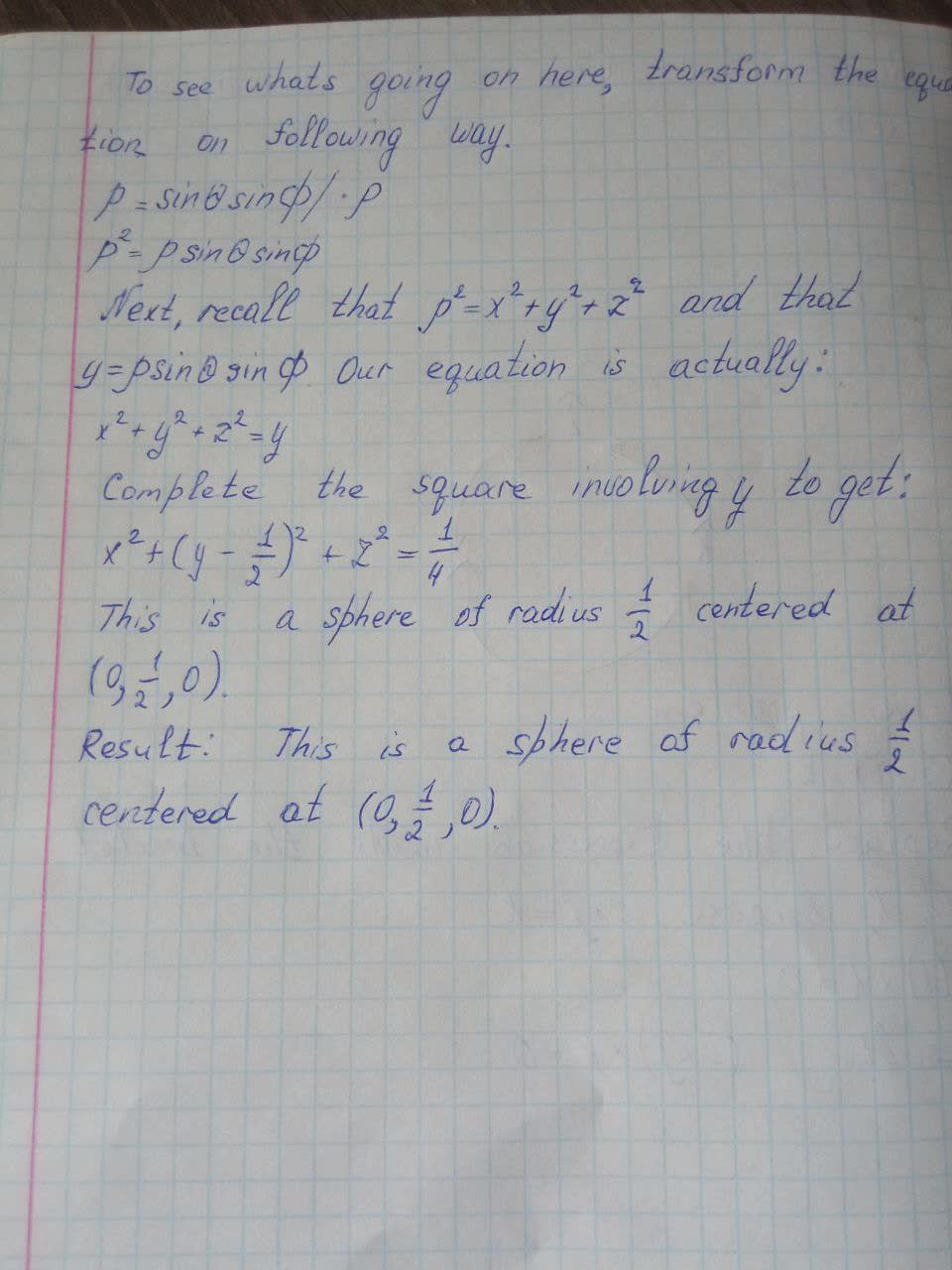Question# Identify the surface whose equation is given. p=\sin\theta\sin\phi

Trigonometric functions
ANSWEREDIdentify the surface whose equation is given. $$\displaystyle{p}={\sin{\theta}}{\sin{\phi}}$$